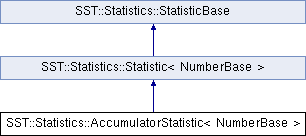SST  7.1.0 StructuralSimulationToolkit
SST::Statistics::AccumulatorStatistic< NumberBase > Class Template Reference

Allows the online gathering of statistical information about a single quantity. More...

`#include <stataccumulator.h>`

Inheritance diagram for SST::Statistics::AccumulatorStatistic< NumberBase >:## Public Member Functions

AccumulatorStatistic (BaseComponent *comp, const std::string &statName, const std::string &statSubId, Params &statParams)

NumberBase getSum ()
Provides the sum of the values presented so far. More...

NumberBase getSumSquared ()
Provides the sum of each value squared presented to the class so far. More...

NumberBase getArithmeticMean ()
Get the arithmetic mean of the values presented so far. More...

NumberBase getVariance ()
Get the variance of the values presented so far. More...

NumberBase getStandardDeviation ()
Get the standard deviation of the values presented so far. More...

uint64_t getCount ()
Get a count of the number of elements presented to the statistics collection so far. More...

void clearStatisticData () override
Inform the Statistic to clear its data.

void registerOutputFields (StatisticOutput *statOutput) override
Called by the system to tell the Statistic to register its output fields. More...

void outputStatisticData (StatisticOutput *statOutput, bool UNUSED(EndOfSimFlag)) override

bool isStatModeSupported (StatisticBase::StatMode_t mode) const overridePublic Member Functions inherited from SST::Statistics::Statistic< NumberBase >
Add data to the Statistic This will call the addData_impl() routine in the derived Statistic.Public Member Functions inherited from SST::Statistics::StatisticBase
void enable ()
Enable Statistic for collections.

void disable ()
Disable Statistic for collections.

virtual void resetCollectionCount ()
Set the current collection count to 0.

virtual void incrementCollectionCount ()
Increment current collection count.

virtual void setCollectionCount (uint64_t newCount)
Set the current collection count to a defined value.

virtual void setCollectionCountLimit (uint64_t newLimit)
Set the collection count limit to a defined value.

void setFlagResetCountOnOutput (bool flag)
Set the Reset Count On Output flag. More...

void setFlagClearDataOnOutput (bool flag)
Set the Clear Data On Output flag. More...

void setFlagOutputAtEndOfSim (bool flag)
Set the Output At End Of Sim flag. More...

const std::string & getCompName () const
Return the Component Name.

const std::string & getStatName () const
Return the Statistic Name.

const std::string & getStatSubId () const
Return the Statistic SubId.

const std::string & getFullStatName () const
Return the full Statistic name of Component.StatName.SubId.

const std::string & getStatTypeName () const
Return the Statistic type name.

const StatisticFieldInfo::fieldType_tgetStatDataType () const
Return the Statistic data type.

const char * getStatDataTypeShortName () const
Return the Statistic data type.

const char * getStatDataTypeFullName () const
Return the Statistic data type.

BaseComponentgetComponent () const
Return a pointer to the parent Component.

bool isEnabled () const
Return the enable status of the Statistic.

bool isOutputEnabled () const
Return the enable status of the Statistic's ability to output data.

uint64_t getCollectionCountLimit () const
Return the collection count limit.

uint64_t getCollectionCount () const
Return the current collection count.

bool getFlagResetCountOnOutput () const
Return the ResetCountOnOutput flag value.

bool getFlagClearDataOnOutput () const
Return the ClearDataOnOutput flag value.

bool getFlagOutputAtEndOfSim () const
Return the OutputAtEndOfSim flag value.

StatMode_t getRegisteredCollectionMode () const
Return the collection mode that is registered.

void delayOutput (const char *delayTime)
Delay the statistic from outputting data for a specified delay time. More...

void delayCollection (const char *delayTime)
Delay the statistic from collecting data for a specified delay time. More...

Indicate that the Statistic is Ready to be used.

virtual bool isNullStatistic () const
Indicate if the Statistic is a NullStatistic.

## Protected Member Functions

Present a new value to the class to be included in the statistics. More...Protected Member Functions inherited from SST::Statistics::Statistic< NumberBase >
Statistic (BaseComponent *comp, const std::string &statName, const std::string &statSubId, Params &statParams)
Construct a Statistic. More...Protected Member Functions inherited from SST::Statistics::StatisticBase
StatisticBase (BaseComponent *comp, const std::string &statName, const std::string &statSubId, Params &statParams)
Construct a StatisticBase. More...

ParamsgetParams ()
Return the Statistic Parameters.

void setStatisticDataType (const StatisticFieldInfo::fieldType_t dataType)
Set the Statistic Data Type.

void setStatisticTypeName (const char *typeName)
Set an optional Statistic Type Name.Public Types inherited from SST::Statistics::StatisticBase
enum  StatMode_t { STAT_MODE_UNDEFINED, STAT_MODE_COUNT, STAT_MODE_PERIODIC }
Statistic collection mode.

## Detailed Description

### template<typename NumberBase> class SST::Statistics::AccumulatorStatistic< NumberBase >

Allows the online gathering of statistical information about a single quantity.

The basic statistics are captured online removing the need to keep a copy of the values of interest.

Template Parameters
 NumberBase A template for the basic numerical type of values

## Member Function Documentation

template<typename NumberBase>
 void SST::Statistics::AccumulatorStatistic< NumberBase >::addData_impl ( NumberBase value )
inlineoverrideprotectedvirtual

Present a new value to the class to be included in the statistics.

Parameters
 value New value to be presented

Implements SST::Statistics::Statistic< NumberBase >.

## ◆ getArithmeticMean()

template<typename NumberBase>
 NumberBase SST::Statistics::AccumulatorStatistic< NumberBase >::getArithmeticMean ( )
inline

Get the arithmetic mean of the values presented so far.

Returns
The arithmetic mean of the values presented so far.

## ◆ getCount()

template<typename NumberBase>
 uint64_t SST::Statistics::AccumulatorStatistic< NumberBase >::getCount ( )
inline

Get a count of the number of elements presented to the statistics collection so far.

Returns
Count the number of values presented to the class.

## ◆ getStandardDeviation()

template<typename NumberBase>
 NumberBase SST::Statistics::AccumulatorStatistic< NumberBase >::getStandardDeviation ( )
inline

Get the standard deviation of the values presented so far.

Returns
The standard deviation of the values presented so far

## ◆ getSum()

template<typename NumberBase>
 NumberBase SST::Statistics::AccumulatorStatistic< NumberBase >::getSum ( )
inline

Provides the sum of the values presented so far.

Returns
The sum of values presented to the class so far.

## ◆ getSumSquared()

template<typename NumberBase>
 NumberBase SST::Statistics::AccumulatorStatistic< NumberBase >::getSumSquared ( )
inline

Provides the sum of each value squared presented to the class so far.

Returns
The sum of squared values presented to the class so far.

## ◆ getVariance()

template<typename NumberBase>
 NumberBase SST::Statistics::AccumulatorStatistic< NumberBase >::getVariance ( )
inline

Get the variance of the values presented so far.

Returns
The variance of the values presented so far

## ◆ registerOutputFields()

template<typename NumberBase>
 void SST::Statistics::AccumulatorStatistic< NumberBase >::registerOutputFields ( StatisticOutput * statOutput )
inlineoverridevirtual

Called by the system to tell the Statistic to register its output fields.

by calling statOutput->registerField(...)

Parameters
 statOutput - Pointer to the statistic output

Implements SST::Statistics::StatisticBase.

The documentation for this class was generated from the following file: